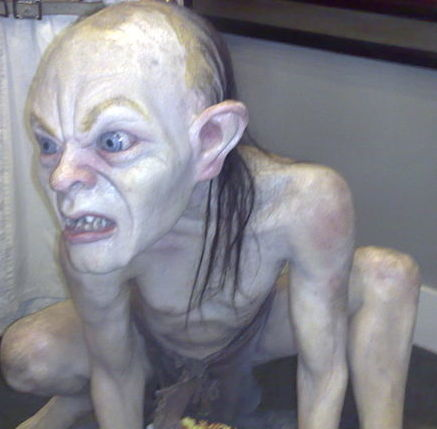# Golomb sequence

Probability Level 4Golomb's sequence is a non-decreasing integer sequence where $a_n$ is the number of times that $n$ occurs in the sequence, starting with $a_1 = 1$, and with the property that for $n > 1$ each $a_n$ is the unique integer which makes it possible to satisfy the condition. The sequence starts off as

$1,2,2,3,3,4,4,4,5,5,5, 6, 6, 6,6, \ldots$

How many times does 50 appear in this sequence?

Image credit: Wikipedia Vic201401

×